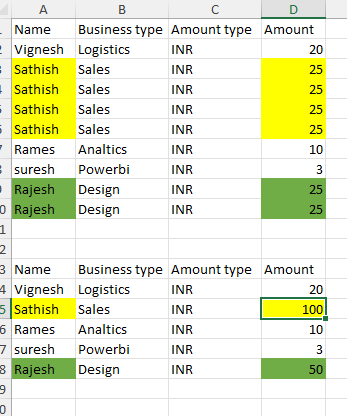# How to Merge cells and sum values if rows are same

I have one excel file and in which that if rows has same values then it has sum amount and merge the two excel rows as one and amount should be summed lets say for example if sathish is repeats in name then that rows should be merged and amount should be added like wise i have to do for that remaining.@sathish_Kumar6

Read the data in to a datatable dt…now use below in the assign activity

Use Build datatable activity with outdt and 4 columns as strings

`outdt = (From d In Dt.AsEnumerable() Group d By k=d("Name").toString.Trim Into grp = Group Let gSum =grp.Sum(function(x) CDBL(x("Amount").ToString).ToString Let ra = New Object(){k,grp.FirstOrDefault(1).ToString, grp.FirstOrDefault(2).ToString,gSum} Select r = outDt.Rows.Add(ra)).CopyToDataTable()`

Cheers

1 Like

Hi,

Hope the following sample helps you.

``````dt = dt.AsEnumerable.GroupBy(Function(r) r("Name").ToString).Select(Function(g) dt.Clone.LoadDataRow({g.Key,g.First()(1),g.First()(2),g.Sum(Function(r) CInt(r("Amount")))},False)).CopyToDataTable
``````

Sample20230520-4L.zip (7.0 KB)

Regards,

1 Like

THank you so much ji @Anil_G

1 Like

Thank so much for your effort reply it means a lot to me

is this linq query ? if so then Where to learn this method properly @Yoichi and @Anil_G

@sathish_Kumar6

Few resources from our mebers

Cheers

1 Like

Thanks a lot @Anil_G

1 Like

hi yoichi,

i have same another excel file but is not working for me so could you please check and let me know.

like if i have linkage id as same then it has to sum qty also please attach excel file fyr
PO-ER-GBA-01398___b54ee9e1-8cc2-42b1-919d-29ff641c4340.xlsx (72.3 KB)

Hi,

Can you check the following sample? (Sequence.xaml)

Sample20230520-4Lv2.zip (22.7 KB)

Regards,

1 Like

Thanks life saver @Yoichi

This topic was automatically closed 3 days after the last reply. New replies are no longer allowed.